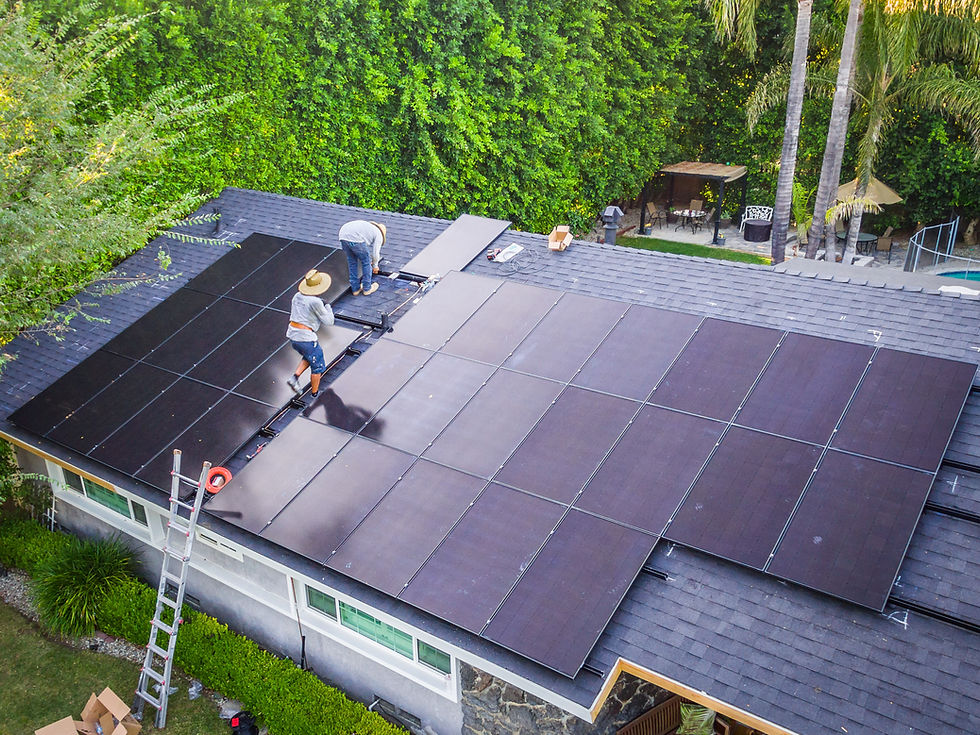top of page# What are Solar Panels?

At the atomic level, a solar panel functions by using the photovoltaic effect to generate electricity. This effect occurs when photons from sunlight strike the surface of a solar panel and knock electrons off the atoms in the panel's semiconductor material, creating a flow of electrons that can be harnessed to generate electricity.

Solar panels are made up of multiple photovoltaic cells, each of which is made up of layers of semiconductor materials, usually silicon. The two layers of silicon in each cell are doped with impurities to create an electric field, which helps to separate the electrons and create a flow of current. When sunlight strikes the cell, it knocks electrons loose from the semiconductor material, creating a flow of electrons across the electric field.

The expected life of a solar panel can vary depending on factors such as the quality of the materials used, the design of the panel, and the environmental conditions it is exposed to. Most solar panels are designed to last for at least 25-30 years, and some can last for 40 years or more with proper maintenance and care.

There are several formulas used to calculate the performance and expected life of a solar panel, including:

The efficiency formula: Efficiency = (power output / irradiance) x 100 This formula is used to calculate the efficiency of a solar panel by dividing the power output by the amount of irradiance (sunlight) hitting the panel and multiplying by 100 to get a percentage.

The degradation rate formula: Degradation rate = (P1 - Pn) / (n x P1) x 100 This formula is used to calculate the degradation rate of a solar panel over time, where P1 is the initial power output, Pn is the power output after n years, and n is the number of years. The formula calculates the percentage decrease in power output over time.

The temperature coefficient formula: Temperature coefficient = (Voc at 25°C - Voc at operating temperature) / (Voc at 25°C x temperature difference) This formula is used to calculate the temperature coefficient of a solar panel, which determines how much the panel's voltage output will decrease as the temperature increases. The formula takes into account the open-circuit voltage (Voc) of the panel at 25°C and the operating temperature of the panel.

Overall, the atomic-level functioning of a solar panel is based on the photovoltaic effect, which generates electricity by knocking electrons off the atoms in the panel's semiconductor material. The expected life of a solar panel can be calculated using formulas such as the efficiency formula, the degradation rate formula, and the temperature

bottom of page